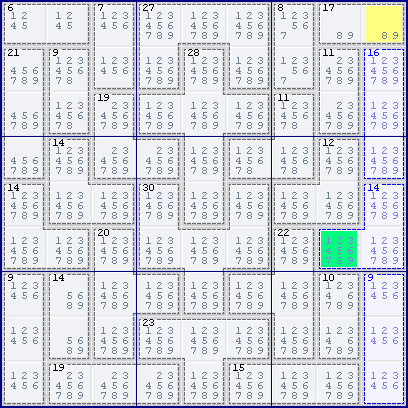Mirrored from Sudopedia, the Free Sudoku Reference Guide

# Innie-outie difference

This is a medium-level solving technique specific to Killer Sudoku. It usually only comes into play with more difficult puzzles.

When a set of cages lies nearly – but not exactly – within one or more houses, sometimes there is a mix of cells that hang outside the house(s) (outies) and cells that are within the house(s) but outside of the cages (innies). By calculating the innie-outie difference (the difference between the sum of the innies and the sum of the outies), we may eliminate candidates from the innie and outie cells.

This technique is usually only useful when few cells are involved, or when the difference between the innies and outies is very large. Typically, in a given puzzle, there are many possible innie-outie differences, most of which yield no useful information; but the patient solver may stumble upon one that helps crack the puzzle.

## Example

This is the starting position of Assassin 7 by Ruud's Weekly Assassin Killer Sudoku.Consider column 9, the innie marked yellow and the outie marked green. Let S be the sum of the cells in column 9 sans the innie (i.e. R23456789C9), I be the value of the innie, and O be the value of the outie. By the 45 rule on the entire column, we have

```S + I = 45
```

On the other hand, the selected cages give

```S + O = 16 + 14 + 9 = 39
```

Subtracting one equation from the other, we get the innie-outie difference

```I - O = 6
```

As the innie I can only be 8 or 9, we find that the outie O can only be 2 or 3. Thus all other candidates (1, 4, 5, 6, 7, 8, 9) can be eliminated from the outie.